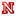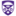Algebraic Geometry Commons™

223 Full-Text Articles 268 Authors 56,862 Downloads69 Institutions

All Articles in Algebraic Geometry

223 full-text articles. Page 7 of 9.

Microrna Expression Data Reveals A Signature Of Kidney Damage Following Ischemia Reperfusion Injury, 2011Tufts University

Microrna Expression Data Reveals A Signature Of Kidney Damage Following Ischemia Reperfusion Injury, Michael D. Shapiro, Jessamyn Bagley, Jeff Latz, Jonathan G. Godwin, Xupeng Ge, Stefan G. Tullius, John Iacomini

GSBS Student Publications

Ischemia reperfusion injury (IRI) is a leading cause of acute kidney injury, a common problem worldwide associated with significant morbidity and mortality. We have recently examined the role of microRNAs (miRs) in renal IRI using expression profiling. Here we conducted mathematical analyses to determine if differential expression of miRs can be used to define a biomarker of renal IRI. Principal component analysis (PCA) was combined with spherical geometry to determine whether samples that underwent renal injury as a result of IRI can be distinguished from controls based on alterations in miR expression using our data set consisting of time series ...

Counting Reducible Composites Of Polynomials, 2011University of Tennessee, Knoxville

Counting Reducible Composites Of Polynomials, Jacob Andrew Ogle

Doctoral Dissertations

This research answers some open questions about the number of reducible translates of a fixed non-constant polynomial over a field. The natural hypothesis to consider is that the base field is algebraically closed in the function field. Since two possible choices for the base field arise, this naturally yields two different hypotheses. In this work, we explicitly relate the two hypotheses arising from this choice. Using the theory of derivations, and specifically an explicit construction of a derivation with a well-understood ring of constants, we can relate the ranks of the two relative-unit-groups involved, both of which are free Abelian ...

Moduli Problems In Derived Noncommutative Geometry, 2011University of Pennsylvania

Moduli Problems In Derived Noncommutative Geometry, Pranav Pandit

Publicly Accessible Penn Dissertations

We study moduli spaces of boundary conditions in 2D topological field theories. To a compactly generated linear infinity-category X, we associate a moduli functor M_X parametrizing compact objects in X. The Barr-Beck-Lurie monadicity theorem allows us to establish the descent properties of M_X, and show that M_X is a derived stack. The Artin-Lurie representability criterion makes manifest the relation between finiteness conditions on X, and the geometricity of M_X. If X is fully dualizable (smooth and proper), then M_X is geometric, recovering a result of Toën-Vaquie from a new perspective. Properness of X does not imply geometricity in general: perfect ...

Homological Projective Duality For Gr(3,6), 2011University of Pennsylvania

Homological Projective Duality For Gr(3,6), Dragos Deliu

Publicly Accessible Penn Dissertations

Homological Projective Duality is a homological extension of the classical no-

tion of projective duality. Constructing the homological projective dual of a variety

allows one to describe semiorthogonal decompositions on the bounded derived cat-

egory of coherent sheaves for all the complete linear sections of the initial variety.

This gives a powerful method to construct decompositions for a big class of varieties,

however examples for which this duality is understood are very few.

In this thesis we investigate the case of Gr(3, 6) with respect to the Plucker

embedding.

The Derived Category And The Singularity Category, 2011University of Pennsylvania

The Derived Category And The Singularity Category, Mehmet U. Isik

Publicly Accessible Penn Dissertations

We prove an equivalence between the derived category of a variety and the equivari- ant/graded singularity category of a corresponding singular variety. The equivalence also holds at the dg level.

2011University of Pennsylvania

The Classification Of Automorphism Groups Of Rational Elliptic Surfaces With Section, Tolga Karayayla

Publicly Accessible Penn Dissertations

In this dissertation, we give a classification of (regular) automorphism groups of relatively minimal rational elliptic surfaces with section over the field ℂ which have non-constant J-maps. The automorphism group Aut(B) of such a surface B is the semi-direct product of its Mordell-Weil group MW(B) and the subgroup Autσ(B) of the automorphisms preserving the zero section σ of the rational elliptic surface B. The configuration of singular fibers on the surface determines the Mordell-Weil group as has been shown by Oguiso and Shioda, and Autσ(B) also depends on the singular fibers. The classification ...

Homology Of Artinian Modules Over Commutative Noetherian Rings, 2011University of Nebraska-Lincoln

Homology Of Artinian Modules Over Commutative Noetherian Rings, Micah J. Leamer

Dissertations, Theses, and Student Research Papers in Mathematics

This work is primarily concerned with the study of artinian modules over commutative noetherian rings.

We start by showing that many of the properties of noetherian modules that make homological methods work seamlessly have analogous properties for artinian modules. We prove many of these properties using Matlis duality and a recent characterization of Matlis reflexive modules. Since Matlis reflexive modules are extensions of noetherian and artinian modules many of the properties that hold for artinian and noetherian modules naturally follow for Matlis reflexive modules and more generally for mini-max modules.

In the last chapter we prove that if the Betti ...

Characteristic Polynomial Of Arrangements And Multiarrangements, 2011The University of Western Ontario

Characteristic Polynomial Of Arrangements And Multiarrangements, Mehdi Garrousian

Electronic Thesis and Dissertation Repository

This thesis is on algebraic and algebraic geometry aspects of complex hyperplane arrangements and multiarrangements. We start by examining the basic properties of the logarithmic modules of all orders such as their freeness, the cdga structure, the local properties and close the first chapter with a multiarrangement version of a theorem due to M. Mustata and H. Schenck.

In the next chapter, we obtain long exact sequences of the logarithmic modules of an arrangement and its deletion-restriction under the tame conditions. We observe how the tame conditions transfer between an arrangement and its deletion-restriction.

In chapter 3, we use some ...

A Characterization Of Dynkin Elements, 2011University of Massachusetts - Amherst

A Characterization Of Dynkin Elements, Pe Gunnells, E Sommers

Paul Gunnells

We give a characterization of the Dynkin elements of a simple Lie algebra. Namely, we prove that one-half of a Dynkin element is the unique point of minimal length in its \$N\$-region. In type \$A_n\$ this translates into a statement about the regions determined by the canonical left Kazhdan-Lusztig cells, which leads to some conjectures in representation theory.

Cohomology Of Congruence Subgroups Of Sl4(Z). Iii, 2011University of Massachusetts - Amherst

Cohomology Of Congruence Subgroups Of Sl4(Z). Iii, A Ash, Pe Gunnells, M Mcconnell

Paul Gunnells

In two previous papers we computed cohomology groups for a range of levels , where is the congruence subgroup of consisting of all matrices with bottom row congruent to mod . In this note we update this earlier work by carrying it out for prime levels up to . This requires new methods in sparse matrix reduction, which are the main focus of the paper. Our computations involve matrices with up to 20 million nonzero entries. We also make two conjectures concerning the contributions to for prime coming from Eisenstein series and Siegel modular forms.

Cohomology Of Congruence Subgroups Of Sl(4, Z) Ii, 2011University of Massachusetts - Amherst

Cohomology Of Congruence Subgroups Of Sl(4, Z) Ii, A Ash, Pe Gunnells, M Mcconnell

Paul Gunnells

In a previous paper  we computed cohomology groups H5(..0(N),C), where ..0(N) is a certain congruence subgroup of SL(4,Z), for a range of levels N. In this note we update this earlier work by extending the range of levels and describe cuspidal cohomology classes and additional boundary phenomena found since the publication of . The cuspidal cohomology classes in this paper are the first cuspforms for GL(4) concretely constructed in terms of Betti cohomology.

Perverse Sheaves On Loop Grassmannians And Langlands Duality, 2011University of Massachusetts - Amherst

Perverse Sheaves On Loop Grassmannians And Langlands Duality, I Mirkovic, K Vilonen

Ivan Mirkovic

No abstract provided.

2011University of Massachusetts - Amherst

Geometric Langlands Duality And Representations Of Algebraic Groups Over Commutative Rings, I Mirkovic, K Vilonen

Ivan Mirkovic

No abstract provided.

On The Locus Of Hodge Classes, 2011University of Massachusetts - Amherst

On The Locus Of Hodge Classes, E Cattani, P Deligne, A Kaplan

Eduardo Cattani

Let S be a nonsingular complex algebraic variety and V a polarized variation of Hodge structure of weight 2p with polarization form Q. Given an integer K, let S(K) be the space of pairs (s, u) with s ∈ S, u ∈ Vs integral of type (p, p), and Q(u, u) ≤ K. We show in Theorem 1.1 that S(K) is an algebraic variety, finite over S. When V is the local system H2p (Xs, Z)/torsion associated with a family of nonsingular projective varieties parametrized by S, the result implies that the locus where a given integral class ...

Frobenius Modules And Hodge Asymptotics, 2011University of Massachusetts - Amherst

Frobenius Modules And Hodge Asymptotics, E Cattani, J Fernandez

Eduardo Cattani

We exhibit a direct correspondence between the potential defining the H1,1 small quantum module structure on the cohomology of a Calabi-Yau manifold and the asymptotic data of the A-model variation of Hodge structure. This is done in the abstract context of polarized variations of Hodge structure and Frobenius modules.

Complete Intersections In Toric Ideals, 2011University of Massachusetts - Amherst

Complete Intersections In Toric Ideals, E Cattani, R Curran, A Dickenstein

Eduardo Cattani

We present examples that show that in dimension higher than one or codimension higher than two, there exist toric ideals such that no binomial ideal contained in and of the same dimension is a complete intersection. This result has important implications in sparse elimination theory and in the study of the Horn system of partial differential equations.

Asymptotic Hodge Theory And Quantum Products, 2011University of Massachusetts - Amherst

Asymptotic Hodge Theory And Quantum Products, E Cattani, Javier Fernandez

Eduardo Cattani

Assuming suitable convergence properties for the Gromov-Witten potential of a Calabi-Yau manifold \$X\$ one may construct a polarized variation of Hodge structure over the complexified K\"ahler cone of \$X\$. In this paper we show that, in the case of fourfolds, there is a correspondence between ``quantum potentials'' and polarized variations of Hodge structures that degenerate to a maximally unipotent boundary point. Under this correspondence, the WDVV equations are seen to be equivalent to the Griffiths' trasversality property of a variation of Hodge structure.

Binomial Residues, 2011University of Massachusetts - Amherst

Binomial Residues, E Cattani, A Dickenstein, B Sturmfels

Eduardo Cattani

A binomial residue is a rational function defined by a hypergeometric integral whose kernel is singular along binomial divisors. Binomial residues provide an integral representation for rational solutions of A-hypergeometric systems of Lawrence type. The space of binomial residues of a given degree, modulo those which are polynomial in some variable, has dimension equal to the Euler characteristic of the matroid associated with A.

2011University of Massachusetts - Amherst

Mixed Lefschetz Theorems And Hodge-Riemann Bilinear Relations, E Cattani

Eduardo Cattani

The Hard Lefschetz Theorem (HLT) and the Hodge–Riemann bilinear relations (HRR) hold in various contexts: they impose restrictions on the cohomology algebra of a smooth compact Kähler manifold; they restrict the local monodromy of a polarized variation of Hodge structure; they impose conditions on the f-vectors of convex polytopes. While the statements of these theorems depend on the choice of a Kähler class, or its analog, there is usually a cone of possible choices. It is then natural to ask whether the HLT and HRR remain true in a mixed context. In this note, we present a unified approach ...

Residues And Resultants, 2011University of Massachusetts - Amherst

Residues And Resultants, E Cattani, Alicia Dickenstein, Bernd Sturmfels

Eduardo Cattani

Resultants, Jacobians and residues are basic invariants of multivariate polynomial systems. We examine their interrelations in the context of toric geometry. The global residue in the torus, studied by Khovanskii, is the sum over local Grothendieck residues at the zeros of \$n\$ Laurent polynomials in \$n\$ variables. Cox introduced the related notion of the toric residue relative to \$n+1\$ divisors on an \$n\$-dimensional toric variety. We establish denominator formulas in terms of sparse resultants for both the toric residue and the global residue in the torus. A byproduct is a determinantal formula for resultants based on Jacobians.